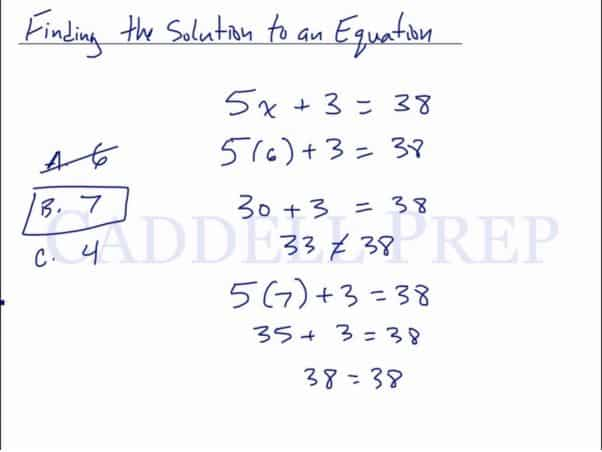In this video, we will be learning how to find the solution to an equation using direct substitution. In this video we will be learning how to declare variables in word problems. After you finish this lesson, view all of our Algebra 1 lessons and practice problems.

Example of How to Find a Solution to an Equation

For what value of x would make this equation true?$3x +1 = 7$       Begin by substituting an answer choice for x

A. 2$3x +1 = 7$

B. 3$3(2) +1 = 7$

C. 4$6 +1 = 7$

D. 5$7 = 7$

Example 1$6x + 4 = 28$. What’s the value of$x$?$A. 3$$B. 5$$C. 4$

Begin by substituting an answer choice for$x$

For$A. 3$$6(3) + 4 = 28$$22\neq28$

For$B. 5$$6(5)+4=28$$34\neq28$

For$C. 4$$6(4)+4=28$$28=28$

So, the correct answer is$B.4$

Example 2$8x -5 = 43$. What’s the value of$x$?$A. 6$$B. 7$$C. 8$

Begin by substituting an answer choice for$x$

For$A. 6$$8(6) -5 = 43$$43=43$

So, the correct answer is$A.6$

Video-Lesson Transcript

Let’s go over finding the solution to an equation.

Basically, we ‘re going to guess and check or use the answer given to us.

This solution is what we’re going to use to know if our answer is correct.

We have:$5x + 3 = 38$. What’s the value of$x$?$A. 6$$B. 7$$C. 4$

So let’s try$A. 6$

we’ll substitue$6$ into$x$ and see if that works.$5(6) + 3 = 38$

Let’s follow the order of operations to see if the equation on the left is equal$38$.$30 + 3 = 38$$33 \neq 38$

So that is not the answer. Let’s try$7$ next.$5(7) + 3 = 38$$35 + 3 = 38$$38 = 38$

So the correct answer is$B. 7$